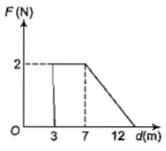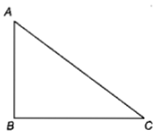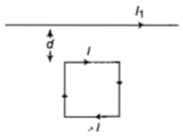An alternating electric field of frequency v, is applied across the dees (radius=R) of a cyclotron that is being used to accelerate protons(mass=m).The operating magnetic field (B) used in the cyclotron and the kinetic energy (K) of the proton beam, produced by it, are given by

(a)B=

(b)B=

(c)B=

(d)B=

Concept Questions :-

Lorentz force
High Yielding Test Series + Question Bank - NEET 2020

Difficulty Level:

A proton carrying 1 MeV kinetic energy is moving in a circular path of radius R in a uniform magnetic field. What should be the energy of an $\mathrm{\alpha }$-particle to describe a circle of the same radius in the same field?

1. 2 MeV                  2. 1 MeV

3. 0.5 MeV               4. 4 MeV

Concept Questions :-

Lorentz force
High Yielding Test Series + Question Bank - NEET 2020

Difficulty Level:

Force F on a particle moving in a straight line varies with distance d as shown in the figure. The work done on the particle during its displacement of 12 m is(a) 21 J                                                  (b) 26 J

(c) 13 J                                                   (d) 18 J

Concept Questions :-

Lorentz force
High Yielding Test Series + Question Bank - NEET 2020

Difficulty Level:

A current-carrying closed loop in the form of a right-angle isosceles triangle ABC is placed in a uniform magnetic field acting along AB. If the magnetic force on the arm BC is F, the force on the arm AC is:1. $\mathbf{-}\mathbf{F}$                                               2. $\mathbf{F}$

3. $\sqrt{2}\mathbf{F}$                                             4. $-\sqrt{2}\mathbf{F}$

Concept Questions :-

Force between current carrying wires
High Yielding Test Series + Question Bank - NEET 2020

Difficulty Level:

A uniform electric field and a uniform magnetic field are acting in the same direction in a certain region. If an electron is projected in the region such that its velocity is pointed along the direction of fields, then the electron :

1. speed will decrease

2. speed will increase

3. will turn towards the left of the direction of motion

4. will turn towards right of direction a motion

Concept Questions :-

Lorentz force
High Yielding Test Series + Question Bank - NEET 2020

Difficulty Level:

A galvanometer of resistance, G is shunted by a resistance S ohm. To keep the main current in the circuit unchanged, the resistance to be put in series with the galvanometer is

(a) $\frac{{S}^{\mathit{2}}}{\left(S+G\right)}$                                             (b) $\frac{SG}{\left(S+G\right)}$

(c) $\frac{{G}^{\mathit{2}}}{\left(S+G\right)}$                                              (d) $\frac{G}{\left(S+G\right)}$

Concept Questions :-

Moving coil galvanometer
High Yielding Test Series + Question Bank - NEET 2020

Difficulty Level:

A square loop, carrying a steady current I, is placed in a horizontal plane near a long straight conductor carrying a steady current ${I}_{\mathit{1}}$ at a distance d from the conductor as shown in figure. The loop will experience(a) a net repulsive force away from the conductor

(b) a net torque acting upward perpendicular to the horizontal plane

(c) a net torque acting downward normal to the horizontal plane

(d) a net attractive force towards the conductor

Concept Questions :-

Lorentz force
High Yielding Test Series + Question Bank - NEET 2020

Difficulty Level:

Charge q is uniformly spread on a thin ring of radius R. The ring rotates about its axis with a uniform frequency f Hz. The magnitude of magnetic induction at the center of the ring is :

(a)                                         (b) $\frac{{\mathrm{\mu }}_{0}\mathrm{q}}{2f\mathrm{R}}$

(c) $\frac{{\mathrm{\mu }}_{0}\mathrm{q}}{2\mathrm{\pi }f\mathrm{R}}$                                       (d)

Concept Questions :-

Magnetic field due to various cases
High Yielding Test Series + Question Bank - NEET 2020

Difficulty Level:

A beam of cathode rays is subjected to crossed Electric (E) and magnetic fields(B). The fields are adjusted such that the beam is not deflected. The specific charge of the cathode rays is given by:

1. $\frac{{B}^{2}}{2V{E}^{2}}$                                             2. $\frac{2V{B}^{2}}{{E}^{2}}$

3. $\frac{2V{E}^{2}}{{B}^{2}}$                                            4. $\frac{{E}^{2}}{2V{B}^{2}}$

(where V is the potential difference between cathode and anode)

Concept Questions :-

Lorentz force
High Yielding Test Series + Question Bank - NEET 2020

Difficulty Level:

A thin ring of radius R metre has charge q coulomb uniformly spread on it.The ring rotates about its axis with a constant frequency of f revolution/s.The value of magnetic induction in  at the centre of the ring is

(a) $\frac{{\mu }_{0}qf}{2\mathrm{\pi R}}$                                          (b)

(c)                                          (d) $\frac{{\mu }_{0}qf}{2R}$

Concept Questions :-

Magnetic field due to various cases